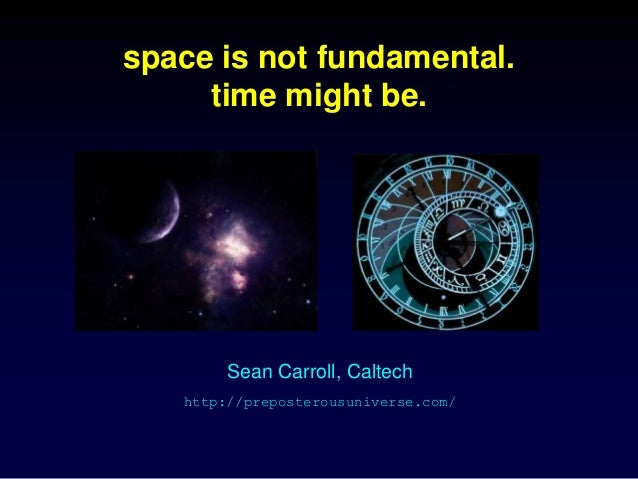Successfully reported this slideshow.Upcoming SlideShare
×

# Against Space

9,303 views

Published on

Space is not fundamental (although time might be). Talk at the 2010 Philosophy of Science Association Meeting, Montreal. By Sean Carroll, http://preposterousuniverse.com/

Published in: Technology
• Full Name
Comment goes here.

Are you sure you want to Yes NoAre you sure you want to  Yes  No

Are you sure you want to  Yes  No

Are you sure you want to  Yes  No

### Against Space

1. 1. space is not fundamental. time might be. Sean Carroll, Caltech http://preposterousuniverse.com/
2. 2. “What is and is not fundamental” is not fundamental. What features will be important ingredients in an ultimate (as yet hypothetical) comprehensive theory of everything. Theories often have very different-looking but equivalent descriptions (e.g. soliton/particle duality). Who is to say what is “fundamental”? But some things are certainly not fundamental; e.g. temperature. Theories using them are not comprehensive. Space is like that.
3. 3. Classical Mechanics Start with a set of coordinates . These obey second-order equations of motion: Specifying the coordinates alone doesn’t determine a solution; need to give and .
4. 4. Coordinates qi and momenta pj. Hamiltonian function H(qi, pj). Hamilton’s equations: Together we have a = {qi, pj}, defining phase space . A single point a(t0) in  defines a unique trajectory. Hamiltonian Mechanics
5. 5. Phase space is a symplectic manifold. A symplectic form  is a closed, invertible 2-form. Trajectories are integral curves of the Hamiltonian vector field,  a(t) Xa
6. 6. The coordinate/momentum distinction is blurred. Conventionally: cotangent bundle T*M = {qi, pi} = phase space  configuration space M, coordinates qi symplectic form  =  dpi  dqi (automatic) Every cotangent bundle is a symplectic manifold, but not every symplectic manifold is a cotangent bundle. Symplecticity is more “fundamental” than coordinate/momentum distinction.
7. 7. Mechanics is invariant under canonical transformations: {q, p}  {Q(q,p), P(q,p)} that leave the form of Hamilton’s equations unchanged. Example: Nothing “fundamental” about which are the coordinates, which are the momenta. Qi = pi , Pj = -qj .
8. 8. Why don’t we live in momentum space? Think of interacting harmonic oscillators. Interactions are local in position, not in momentum. Better: position is the thing in which interactions are local.
9. 9. Quantum mechanics States are rays in Hilbert space: |. Evolution is governed by the Schrödinger equation: Energy eigenbasis: Dynamics are defined by the eigenvalues {En}, the spectrum of the Hamiltonian.
10. 10. Where is “space” in the quantum state? We can define a position operator with eigenstates in terms of which the state is But we don’t have to; momentum also works. These are related by Fourier transform,
11. 11. Or other bases, e.g. creation/annihilation operators for a simple harmonic oscillator. Here, These operators raise and lower energy eigenstates:
12. 12. Entanglement For a generic multiparticle state |, The wave function is not a function of space, but of many copies of space. Things don’t happen in “space”; they happen in Hilbert space. Again, it’s locality of interactions that tempts us to speak otherwise.
13. 13. Quantum Field Theory QFT would seem to deeply privilege “space”; the Hamiltonian is an integral over space. But why? Interactions are local in space: not in momentum:
14. 14. Gravity Consider a compact dimension on a circle. R A scalar field  can be decomposed into Kaluza-Klein modes with energies From the higher-dimensional perspective, these modes comprise a tower of massive states. Conversely: if every field has such a tower, that implies an extra dimension.
15. 15. M-theory’s 11th dimension Witten 1995: there are supersymmetric particle multiplets in Type IIA string theory with masses that depend on the coupling  as Small : states are heavy and decouple. Large : Kaluza-Klein tower, as if an extra dimension. Q: How many dimensions are there in string theory? A: It depends. x11  10 dimensional IIA string theory 11 dimensional supergravity
16. 16. T-duality: string theory on a small circle is equivalent to string theory on a big circle. Momentum/winding duality. Mirror symmetries: IIA string theory on one Calabi-Yau manifold equals IIB string theory on another one. These are gauge symmetries; exact equivalence. No such thing as the “true” compactification.
17. 17. R Holography Maximum entropy inside a region of space doesn’t go as R3, the volume, but as R2, the area. Discovered in the context of black holes, but believed to be more general. Significance: The world is not made of separate degrees of freedom at each point in space. Emergent space isn’t just a matter of discreteness.
18. 18. Maldacena, 1997: quantum gravity (string theory) on five-dimensional anti-de Sitter space times a five-sphere is equivalent to a conformal field theory without gravity on the four-dimensional boundary. “The spacetime one is in” is not unambiguously defined. 10 dimensions AdS5 x S5 4-dimensional Minkowski space AdS/CFT
19. 19. • QM, states, time, & the Schrödinger equation: Space somehow recovered from |. • QM, states, & the Wheeler-de Witt equation: Space and time recovered from |. • A generalization of, or replacement for, QM. What might be fundamental?
20. 20. Closing ruminations • Space/coordinates are picked out by the specific Hamiltonian of the world, not by the structure of our theories. • Investigations of quantum gravity provide strong evidence that space is emergent, and in a deeper way than local discreteness. Degrees of freedom are not local. • Unwarranted speculation: trying to understand the early universe will help us understand the role of space & time.